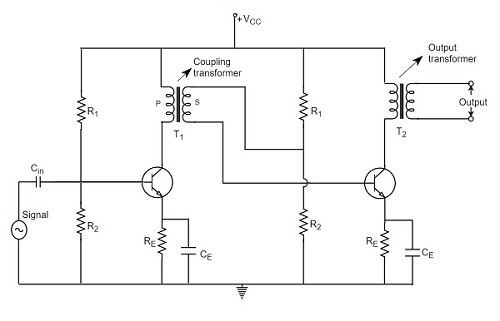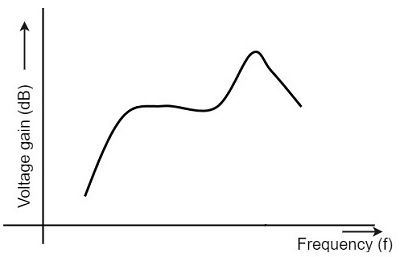# Transformer Coupled Amplifier

Advertisements

We have observed that the main drawback of RC coupled amplifier is that the effective load resistance gets reduced. This is because, the input impedance of an amplifier is low, while its output impedance is high.

When they are coupled to make a multistage amplifier, the high output impedance of one stage comes in parallel with the low input impedance of next stage. Hence, effective load resistance is decreased. This problem can be overcome by a transformer coupled amplifier.

In a transformer-coupled amplifier, the stages of amplifier are coupled using a transformer. Let us go into the constructional and operational details of a transformer coupled amplifier.

## Construction of Transformer Coupled Amplifier

The amplifier circuit in which, the previous stage is connected to the next stage using a coupling transformer, is called as Transformer coupled amplifier.

The coupling transformer T1 is used to feed the output of 1st stage to the input of 2nd stage. The collector load is replaced by the primary winding of the transformer. The secondary winding is connected between the potential divider and the base of 2nd stage, which provides the input to the 2nd stage. Instead of coupling capacitor like in RC coupled amplifier, a transformer is used for coupling any two stages, in the transformer coupled amplifier circuit.

The figure below shows the circuit diagram of transformer coupled amplifier.The potential divider network R1 and R2 and the resistor Re together form the biasing and stabilization network. The emitter by-pass capacitor Ce offers a low reactance path to the signal. The resistor RL is used as a load impedance. The input capacitor Cin present at the initial stage of the amplifier couples AC signal to the base of the transistor. The capacitor CC is the coupling capacitor that connects two stages and prevents DC interference between the stages and controls the shift of operating point.

## Operation of Transformer Coupled Amplifier

When an AC signal is applied to the input of the base of the first transistor then it gets amplified by the transistor and appears at the collector to which the primary of the transformer is connected.

The transformer which is used as a coupling device in this circuit has the property of impedance changing, which means the low resistance of a stage (or load) can be reflected as a high load resistance to the previous stage. Hence the voltage at the primary is transferred according to the turns ratio of the secondary winding of the transformer.

This transformer coupling provides good impedance matching between the stages of amplifier. The transformer coupled amplifier is generally used for power amplification.

## Frequency Response of Transformer Coupled Amplifier

The figure below shows the frequency response of a transformer coupled amplifier. The gain of the amplifier is constant only for a small range of frequencies. The output voltage is equal to the collector current multiplied by the reactance of primary.At low frequencies, the reactance of primary begins to fall, resulting in decreased gain. At high frequencies, the capacitance between turns of windings acts as a bypass condenser to reduce the output voltage and hence gain.

So, the amplification of audio signals will not be proportionate and some distortion will also get introduced, which is called as Frequency distortion.

## Advantages of Transformer Coupled Amplifier

The following are the advantages of a transformer coupled amplifier −

• An excellent impedance matching is provided.
• Gain achieved is higher.
• There will be no power loss in collector and base resistors.
• Efficient in operation.

## Disadvantages of Transformer Coupled Amplifier

The following are the disadvantages of a transformer coupled amplifier −

• Though the gain is high, it varies considerably with frequency. Hence a poor frequency response.

• Frequency distortion is higher.

• Transformers tend to produce hum noise.

• Transformers are bulky and costly.

### Applications

The following are the applications of a transformer coupled amplifier −

• Mostly used for impedance matching purposes.
• Used for Power amplification.
• Used in applications where maximum power transfer is needed.
Advertisements